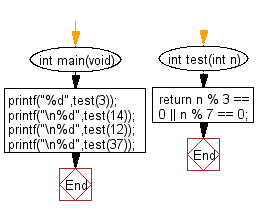﻿ C Program: Test a positive number is multiple of 3 or 7# C Exercises: Check whether a given positive number is a multiple of 3 or a multiple of 7

## C-programming basic algorithm: Exercise-5 with Solution

Write a C program to check whether a given positive number is a multiple of 3 or a multiple of 7.

C Code:

``````#include <stdio.h>
#include <stdlib.h>
int main(void){
printf("%d",test(3));
printf("\n%d",test(14));
printf("\n%d",test(12));
printf("\n%d",test(37));
}
int test(int n)
{
return n % 3 == 0 || n % 7 == 0;
}
``````

Sample Output:

```1
1
1
0
```

Pictorial Presentation:Flowchart:C Programming Code Editor:

What is the difficulty level of this exercise?

Test your Programming skills with w3resource's quiz.

﻿

## C Programming: Tips of the Day

Why doesn't a+++++b work?

printf("%d",a+++++b); is interpreted as (a++)++ + b according to the Maximal Munch Rule!.

++ (postfix) doesn't evaluate to an lvalue but it requires its operand to be an lvalue.

! 6.4/4 says the next preprocessing token is the longest sequence of characters that could constitute a preprocessing token"

Ref : https://bit.ly/3fdldUT